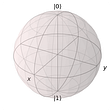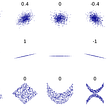# Q-Intro: Grover Iterator

In the previous, introductory article we have discussed about the amplitude amplifier and detailed example.

In this introduction article, we discuss about what shall be the maximum and minimum threshold iterations required to obtain the probability for the chosen element over the distribution.

Let us consider, we want to choose one element out of N elements quantumly.

We define the states in the similar notation as we defined in the previous article: chosen represents the selected element, others represent the remaining elements as shown below.

We walk through the circuit as shown below,

at point 2, the wave function is as shown below,

with the above consider notation it is easy to see that wave function is a simple summation of both type of elements as shown below

with the further simplification we can rewrite as

by representing this in the 2-dimensional graph as shown below

At point 3, passing through the Oracle the chosen element shall be phase inverted as shown below

by representing this in the 2-dimensional graph |𝜓 chosen⟩ in negative axis as shown below

At point 4, after passing through the inversion about the mean operator the state shall be as shown below

Where M is the Inversion about mean operator and Uf is the oracle, and considering U𝑓|𝜓⟩= |𝜙⟩

re writing the wave function at point 4 as shown below,

by representing this in the 2-dimensional graph with reflection around |𝜓⟩ as shown below,

Therefore, we observe that (2|𝜓⟩⟨𝜓|−𝐼)Uf is a rotation.

let us pick cos𝜃/2 and sin𝜃/2 from the wave function, as shown below

then the wave function can be rewritten as shown below,

Goal: is to bring the “MUf|𝜓⟩” as close as possible to vertical axis, we need to figure out how many rotations it takes to reach to the vertical axis approximately.

After applying one complete iteration for the given circuit we have “MUf|𝜓⟩”

we can simplify as follows,

after passing through the oracle

after passing through the Inversion about Mean M operator as shown below

multiplying the terms gives as follows

simplifying the terms further bringing common terms together as shown below

Replacing with the above conditions (fig condition1 and fig condition 2) in the above term( fig term1), we get as shown below

applying the formulae; sin²𝜃 + cos²𝜃 = 1 replacing cos²𝜃 as 1-sin²𝜃 and sin²𝜃 as 1-cos²𝜃 as shown below

after further simplifying

applying the formulae; cos 3𝜃 = 4cos³𝜃 -3cos𝜃 and sin3𝜃 = 3sin𝜃 -4sin³𝜃 as shown below

After applying “t” iteration for the given circuit as shown below

for the chosen element the result shall be as shown below

other terms get cancel or becomes zero and chosen terms becomes 1, we can see the same in the graph we have discussed above, which gives the following condition

replacing with the assumption we made earlier gives as follows

the value of number of rotations having a maximum threshold as follows:

Instead of one element, we want to choose K elements out of N elements quantumly, then the upper limit shall be as shown below.

Observations:

1. for K = 1 following is the data collected

where,

sqrt(N) iteration means upper nearest sqrt(N) integer value.

sqrt(N) probabilities means probabilities observed for the nearest sqrt(N) integer iteration.

best iteration means the iteration where we have best probability for chosen value (note: this value is chosen below sqrt(N)).

best probabilities means probabilities observed for the best iteration.

code snippet used to collect the data is as shown above

we can observe the max and min limits as following

greater than 50% but less than 70% for up to n = 30(unable to compute for values greater than 30 due to inf problem)

2. “MUf” is nothing but Grover Operator which shall be iterate for t times.

Update: (19/feb/2022) updated with missing parts of derivation(fig condition1 and fig condition 2).

References: qiskit textbook, cirq tutorials, IITM course by (@Prabha M, Anil Prabhakar), Qubit by Qubit(The Coding School) course, Textbook by Noson S, Wikipedia, Textbook by Phillip Kaye, stack exchange and open source materials.

--

--

--

## More from Anonymousket

Love podcasts or audiobooks? Learn on the go with our new app.

## Blocking I/O and non-blocking I/O## Creating MineField In Unity (Part 1)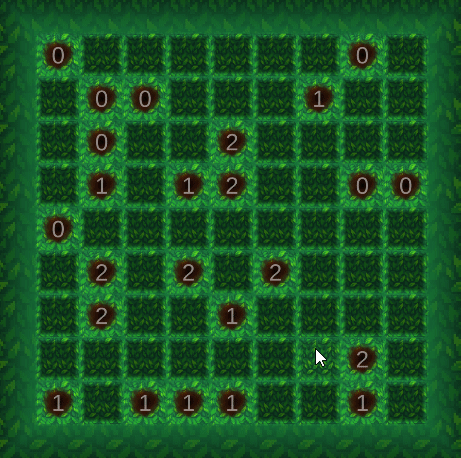## Odoo Zoom Integration — Simplifying Meetings Management## An Introduction to Microsoft Power Platform## Authenticating ASP.NET Core Web Application with Azure AD B2C using User Flows## Leveraging the Denodo Platform to Streamline Our Data Infrastructure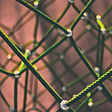## How did I ace my algorithm and data structure, again!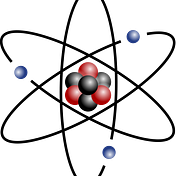## Quantum Algo: Grover Algorithm